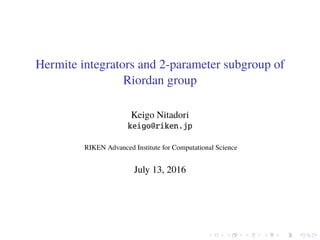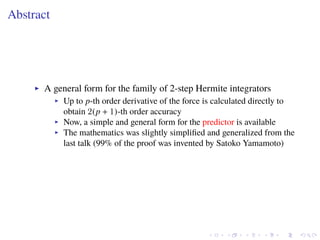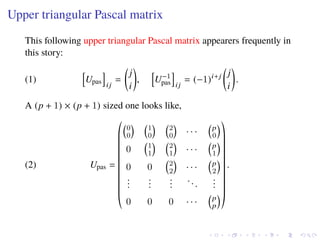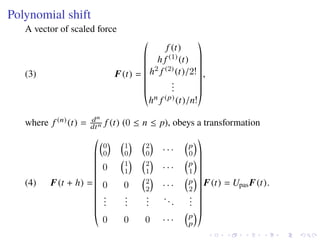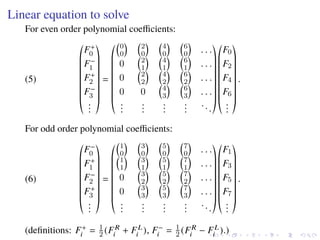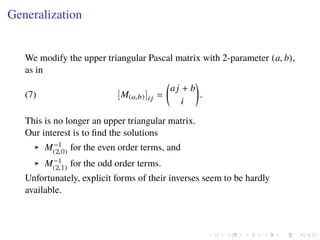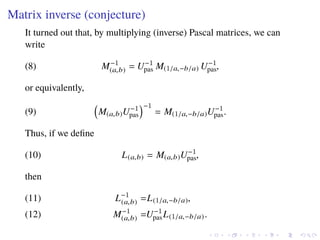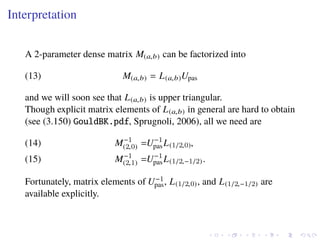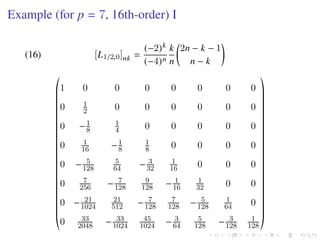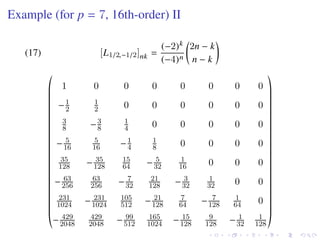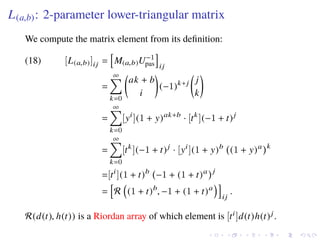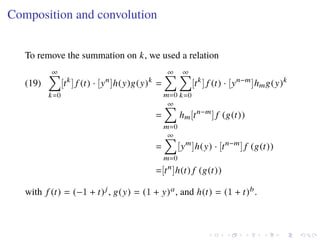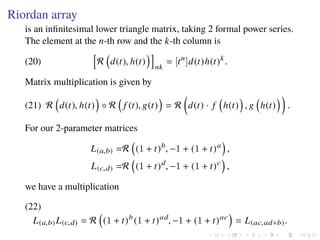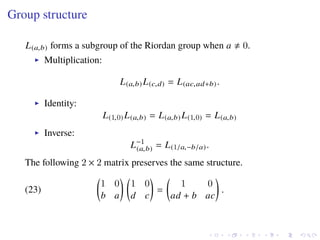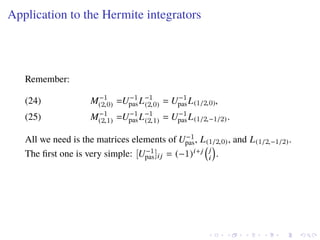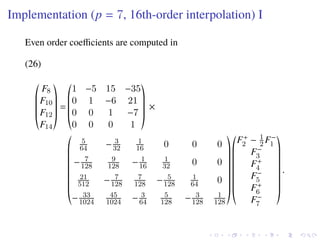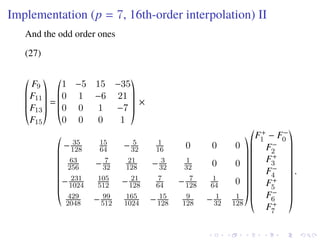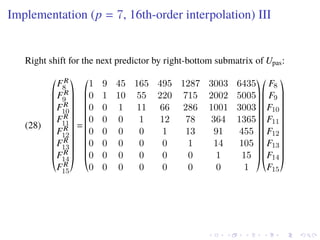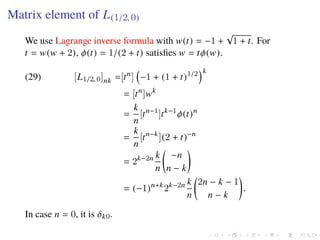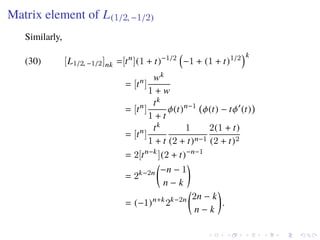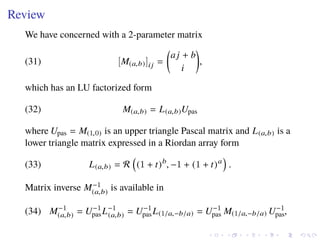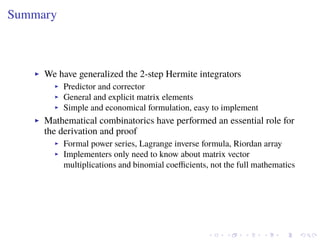1 de 22

### Hermite integrators and 2-parameter subgroup of Riordan group

1. Hermite integrators and 2-parameter subgroup of Riordan group Keigo Nitadori keigo@riken.jp RIKEN Advanced Institute for Computational Science July 13, 2016
2. Abstract A general form for the family of 2-step Hermite integrators Up to p-th order derivative of the force is calculated directly to obtain 2(p + 1)-th order accuracy Now, a simple and general form for the predictor is available The mathematics was slightly simpliﬁed and generalized from the last talk (99% of the proof was invented by Satoko Yamamoto)
3. Upper triangular Pascal matrix This following upper triangular Pascal matrix appearers frequently in this story: (1) Upas ij = j i , U−1 pas ij = (−1)i+j j i . A (p + 1) × (p + 1) sized one looks like, (2) Upas = 0 0 1 0 2 0 · · · p 0 0 1 1 2 1 · · · p 1 0 0 2 2 · · · p 2 ... ... ... ... ... 0 0 0 · · · p p .
4. Polynomial shift A vector of scaled force (3) F(t) = f (t) h f (1) (t) h2 f (2) (t)/2! ... hn f (p) (t)/n! , where f (n) (t) = dn dtn f (t) (0 ≤ n ≤ p), obeys a transformation F(t + h) = 0 0 1 0 2 0 · · · p 0 0 1 1 2 1 · · · p 1 0 0 2 2 · · · p 2 ... ... ... ... ... 0 0 0 · · · p p F(t) = UpasF(t).(4)
5. Linear equation to solve For even order polynomial coeﬃcients: (5) F+ 0 F− 1 F+ 2 F− 3 ... = 0 0 2 0 4 0 6 0 . . . 0 2 1 4 1 6 1 . . . 0 2 2 4 2 6 2 . . . 0 0 4 3 6 3 . . . ... ... ... ... ... F0 F2 F4 F6 ... . For odd order polynomial coeﬃcients: (6) F− 0 F+ 1 F− 2 F+ 3 ... = 1 0 3 0 5 0 7 0 . . . 1 1 3 1 5 1 7 1 . . . 0 3 2 5 2 7 2 . . . 0 3 3 5 3 7 3 . . . ... ... ... ... ... F1 F3 F5 F7 ... . (deﬁnitions: F+ i = 1 2 (FR i + FL i ), F− i = 1 2 (FR i − FL i ).)
6. Generalization We modify the upper triangular Pascal matrix with 2-parameter (a, b), as in (7) [M(a,b)]ij = aj + b i . This is no longer an upper triangular matrix. Our interest is to ﬁnd the solutions M−1 (2,0) for the even order terms, and M−1 (2,1) for the odd order terms. Unfortunately, explicit forms of their inverses seem to be hardly available.
7. Matrix inverse (conjecture) It turned out that, by multiplying (inverse) Pascal matrices, we can write (8) M−1 (a,b) = U−1 pas M(1/a,−b/a) U−1 pas, or equivalently, (9) M(a,b)U−1 pas −1 = M(1/a,−b/a)U−1 pas. Thus, if we deﬁne (10) L(a,b) = M(a,b)U−1 pas, then L−1 (a,b) =L(1/a,−b/a),(11) M−1 (a,b) =U−1 pasL(1/a,−b/a).(12)
8. Interpretation A 2-parameter dense matrix M(a,b) can be factorized into (13) M(a,b) = L(a,b)Upas and we will soon see that L(a,b) is upper triangular. Though explicit matrix elements of L(a,b) in general are hard to obtain (see (3.150) GouldBK.pdf, Sprugnoli, 2006), all we need are M−1 (2,0) =U−1 pasL(1/2,0),(14) M−1 (2,1) =U−1 pasL(1/2,−1/2).(15) Fortunately, matrix elements of U−1 pas, L(1/2,0), and L(1/2,−1/2) are available explicitly.
9. Example (for p = 7, 16th-order) I (16) [L1/2,0]nk = (−2)k (−4)n k n 2n − k − 1 n − k 1 0 0 0 0 0 0 0 0 1 2 0 0 0 0 0 0 0 −1 8 1 4 0 0 0 0 0 0 1 16 −1 8 1 8 0 0 0 0 0 − 5 128 5 64 − 3 32 1 16 0 0 0 0 7 256 − 7 128 9 128 − 1 16 1 32 0 0 0 − 21 1024 21 512 − 7 128 7 128 − 5 128 1 64 0 0 33 2048 − 33 1024 45 1024 − 3 64 5 128 − 3 128 1 128
10. Example (for p = 7, 16th-order) II (17) [L1/2,−1/2]nk = (−2)k (−4)n 2n − k n − k 1 0 0 0 0 0 0 0 −1 2 1 2 0 0 0 0 0 0 3 8 −3 8 1 4 0 0 0 0 0 − 5 16 5 16 −1 4 1 8 0 0 0 0 35 128 − 35 128 15 64 − 5 32 1 16 0 0 0 − 63 256 63 256 − 7 32 21 128 − 3 32 1 32 0 0 231 1024 − 231 1024 105 512 − 21 128 7 64 − 7 128 1 64 0 − 429 2048 429 2048 − 99 512 165 1024 − 15 128 9 128 − 1 32 1 128
11. L(a,b): 2-parameter lower-triangular matrix We compute the matrix element from its deﬁnition: [L(a,b)]ij = M(a,b)U−1 pas ij (18) = ∞ k=0 ak + b i (−1)k+j j k = ∞ k=0 [yi ](1 + y)ak+b · [tk ](−1 + t)j = ∞ k=0 [tk ](−1 + t)j · [yi ](1 + y)b (1 + y)a k =[ti ](1 + t)b −1 + (1 + t)a j = R (1 + t)b , −1 + (1 + t)a ij . R(d(t), h(t)) is a Riordan array of which element is [ti]d(t)h(t)j.
12. Composition and convolution To remove the summation on k, we used a relation ∞ k=0 [tk ] f (t) · [yn ]h(y)g(y)k = ∞ m=0 ∞ k=0 [tk ] f (t) · [yn−m ]hmg(y)k (19) = ∞ m=0 hm[tn−m ] f (g(t)) = ∞ m=0 [ym ]h(y) · [tn−m ] f (g(t)) =[tn ]h(t) f (g(t)) with f (t) = (−1 + t)j, g(y) = (1 + y)a, and h(t) = (1 + t)b.
13. Riordan array is an inﬁnitesimal lower triangle matrix, taking 2 formal power series. The element at the n-th row and the k-th column is (20) R d(t), h(t) nk = [tn ]d(t)h(t)k . Matrix multiplication is given by R d(t), h(t) ◦ R f (t), g(t) = R d(t) · f h(t) , g h(t) .(21) For our 2-parameter matrices L(a,b) =R (1 + t)b , −1 + (1 + t)a , L(c,d) =R (1 + t)d , −1 + (1 + t)c , we have a multiplication L(a,b) L(c,d) = R (1 + t)b (1 + t)ad , −1 + (1 + t)ac = L(ac,ad+b). (22)
14. Group structure L(a,b) forms a subgroup of the Riordan group when a 0. Multiplication: L(a,b) L(c,d) = L(ac,ad+b). Identity: L(1,0) L(a,b) = L(a,b) L(1,0) = L(a,b) Inverse: L−1 (a,b) = L(1/a,−b/a). The following 2 × 2 matrix preserves the same structure. (23) 1 0 b a 1 0 d c = 1 0 ad + b ac .
15. Application to the Hermite integrators Remember: M−1 (2,0) =U−1 pasL−1 (2,0) = U−1 pasL(1/2,0),(24) M−1 (2,1) =U−1 pasL−1 (2,1) = U−1 pasL(1/2,−1/2).(25) All we need is the matrices elements of U−1 pas, L(1/2,0), and L(1/2,−1/2). The ﬁrst one is very simple: [U−1 pas]ij = (−1)i+j j i .
16. Implementation (p = 7, 16th-order interpolation) I Even order coeﬃcients are computed in F8 F10 F12 F14 = 1 −5 15 −35 0 1 −6 21 0 0 1 −7 0 0 0 1 × (26) 5 64 − 3 32 1 16 0 0 0 − 7 128 9 128 − 1 16 1 32 0 0 21 512 − 7 128 7 128 − 5 128 1 64 0 − 33 1024 45 1024 − 3 64 5 128 − 3 128 1 128 F+ 2 − 1 2 F− 1 F− 3 F+ 4 F− 5 F+ 6 F− 7 .
17. Implementation (p = 7, 16th-order interpolation) II And the odd order ones F9 F11 F13 F15 = 1 −5 15 −35 0 1 −6 21 0 0 1 −7 0 0 0 1 × (27) − 35 128 15 64 − 5 32 1 16 0 0 0 63 256 − 7 32 21 128 − 3 32 1 32 0 0 − 231 1024 105 512 − 21 128 7 64 − 7 128 1 64 0 429 2048 − 99 512 165 1024 − 15 128 9 128 − 1 32 1 128 F+ 1 − F− 0 F− 2 F+ 3 F− 4 F+ 5 F− 6 F+ 7 .
18. Implementation (p = 7, 16th-order interpolation) III Right shift for the next predictor by right-bottom submatrix of Upas: FR 8 FR 9 FR 10 FR 11 FR 12 FR 13 FR 14 FR 15 = 1 9 45 165 495 1287 3003 6435 0 1 10 55 220 715 2002 5005 0 0 1 11 66 286 1001 3003 0 0 0 1 12 78 364 1365 0 0 0 0 1 13 91 455 0 0 0 0 0 1 14 105 0 0 0 0 0 0 1 15 0 0 0 0 0 0 0 1 F8 F9 F10 F11 F12 F13 F14 F15 (28)
19. Matrix element of L(1/2, 0) We use Lagrange inverse formula with w(t) = −1 + √ 1 + t. For t = w(w + 2), φ(t) = 1/(2 + t) satisﬁes w = tφ(w). [L1/2, 0]nk =[tn ] −1 + (1 + t)1/2 k (29) = [tn ]wk = k n [tn−1 ]tk−1 φ(t)n = k n [tn−k ](2 + t)−n = 2k−2n k n −n n − k = (−1)n+k 2k−2n k n 2n − k − 1 n − k . In case n = 0, it is δk0.
20. Matrix element of L(1/2, −1/2) Similarly, [L1/2, −1/2]nk =[tn ](1 + t)−1/2 −1 + (1 + t)1/2 k (30) = [tn ] wk 1 + w = [tn ] tk 1 + t φ(t)n−1 φ(t) − tφ (t) = [tn ] tk 1 + t 1 (2 + t)n−1 2(1 + t) (2 + t)2 = 2[tn−k ](2 + t)−n−1 = 2k−2n −n − 1 n − k = (−1)n+k 2k−2n 2n − k n − k .
21. Review We have concerned with a 2-parameter matrix (31) [M(a,b)]ij = aj + b i , which has an LU factorized form (32) M(a,b) = L(a,b)Upas where Upas = M(1,0) is an upper triangle Pascal matrix and L(a,b) is a lower triangle matrix expressed in a Riordan array form (33) L(a,b) = R (1 + t)b , −1 + (1 + t)a . Matrix inverse M−1 (a,b) is available in (34) M−1 (a,b) = U−1 pasL−1 (a,b) = U−1 pasL(1/a,−b/a) = U−1 pas M(1/a,−b/a) U−1 pas,
22. Summary We have generalized the 2-step Hermite integrators Predictor and corrector General and explicit matrix elements Simple and economical formulation, easy to implement Mathematical combinatorics have performed an essential role for the derivation and proof Formal power series, Lagrange inverse formula, Riordan array Implementers only need to know about matrix vector multiplications and binomial coeﬃcients, not the full mathematics